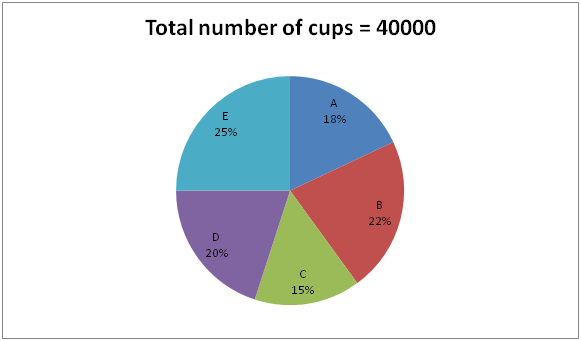# CWC/FCI Prelims 2019 – Quantitative Aptitude Questions (Day-03)

Dear Readers, Exam Race for the Year 2019 has already started, To enrich your preparation here we are providing new series of Practice Questions on Quantitative Aptitude – Section for CWC/FCI Exam. Aspirants, practice these questions on a regular basis to improve your score in aptitude section. Start your effective preparation from the right beginning to get success in upcoming CWC/FCI Exam.

[WpProQuiz 5406]

Directions (Q. 1 – 5): What approximate value should come in place of question mark (?) in the following questions?

1) (32/7) of [8955.21 – 2368.13] + 234.5 =? + 3429.87

a) 31560

b) 22440

c) 26920

d) 34280

e) 17650

2) 24 % of 849 + 56 % of 1299 – 456.23 ÷ 4 =? + (8.11)2

a) 686

b) 612

c) 575

d) 438

e) 754

3) 17 % of ? – 2054 ÷ 12.78 + 222 ÷ 3.01 = 57

a) 1700

b) 2000

c) 2200

d) 1300

e) 2500

4) 5 (¼) + 3 (5/8) – 4 (2/3) + 2 (½) =? – 4 (3/4)

a) 20

b) 6

c) 24

d) 12

e) 30

5) [(46.04)2 ÷ 23 × 6.11] ÷ 12 = 2.99 ×? – 788 ÷ 3

a) 158

b) 103

c) 215

d) 267

e) 99

Directions (6 – 10): Study the following information carefully and answer the questions given below.

The following pie chart shows the total number of plastic cups manufactured by five different companies in a certain week.6) If 12 % and 8 % of plastic cups are defective which was manufactured by the companies A and C respectively, then find the difference between the total number of non – defective cups of company A to that of C?

a) 978

b) 724

c) 816

d) 852

e) None of these

7) The price per plastic cup of company B is Rs. 1.50. Find the total cost of all the cups in the company B, if 5 % of cups are defective (Defective cups are rejected by the company itself)?

a) Rs. 15780

b) Rs. 12540

c) Rs. 13450

d) Rs. 17890

e) None of these

8) Find the average number of plastic cups manufactured by the company C, D and E together?

a) 7000

b) 6500

c) 9000

d) 8000

e) None of these

9) If 42 % of the plastic cups manufactured by the company D is exported to the foreign countries, then find the total number of plastic cups is exported to the foreign countries by company D?

a) 2190

b) 2540

c) 3780

d) 3360

e) None of these

10) Total number of plastic cups manufactured by company A is what percentage of total number of plastic cups manufactured by company E?

a) 72 %

b) 84 %

c) 95 %

d) 66 %

e) None of these

Direction (1-5) :

(32/7) of [8955 – 2368] + 235 = x + 3430

(32/7)*(6587) + 235 – 3430 = x

X = 30112 + 235 – 3430 = 26917

X = 26920

24 % of 850 + 56 % of 1300 – 456 ÷ 4 = x + 82

(24/100)*850 + (56/100)*1300 – (456/4) – 64 = x

204 + 728 – 114 – 64 = x

X = 754

(17/100)*x – (2054/13) + (222/3) = 57

(17/100)*x = 57 + 158 + 74

(17/100)*x = 289

X = 1700

5 (¼) + 3 (5/8) – 4 (2/3) + 2 (½) + 4 (¾) = x

X = 5 + 4 – 5 + 3 + 5 = 12

[(46)2 ÷ 23 × 6] ÷ 12 = 3x – 789 ÷ 3

(46*46*6)/(23*12) = 3x – (789/3)

46 + 263 = 3x

3x = 309

X = 103

Direction (6-10) :

The total number of non – defective cups of company A

= > 40000*(18/100)*(88/100)

= > 6336

The total number of non – defective cups of company C

= > 40000*(15/100)*(92/100)

= > 5520

Required difference = 6336 – 5520 = 816

Total number of non – defective cups in company B

= > 40000*(22/100)*(95/100)

= > 8360

The price per plastic cup in company B = Rs. 1.50

Total cost = 8360*1.50 = Rs. 12540

The average number of plastic cups manufactured by the company C, D and E together

= > (15 + 20 + 25)*(40000/300)

= > 8000

The total number of plastic cups is exported to foreign countries by company D

= > 40000*(20/100)*(42/100)

= > 3360